# 11. PTM2, C4 – The ADF test

## 11.1 – Co-Integration of two-time series

I guess this chapter will get a little complex. We would be skimming the surface of some higher order statistical theory. I will try my best and stick to practical stuff and avoid all the fluff. I’ll try and explain these things from a trading point of view, but I’m afraid, some amount of theory will be necessary for you to know.

Given the path ahead I think it is necessary to re-rack our learnings so far and put some order to it. Hence let me just summarize our journey so far –

1. Starting from Chapter 1 to 7, we discussed a very basic version of a pair trade. We discussed this simply to lay out a strong foundation for the higher order pair trading technique, which is generally known as the relative value trade
2. The relative value trade requires the use of linear regression
3. In linear regression, we regress an independent variable, X against a dependent variable Y.
4. When we regress – some of the outputs that are of interest are the intercept, slope, residuals, standard error, and the standard error of the intercept
5. The decision to classify a stock as dependent and independent really depends on the error ratio.
6. We calculate the error ratio by interchanging both X and Y. The one which offers the lowest error ratio will define which stock is X and which on as Y.

I hope you have read and understood everything that we have discussed up to this point. If not, I’d suggest you read the chapters again, get clarity, and then proceed.

Recollect, in the previous chapter, we discussed the residuals. In fact, I also mentioned that the bulk of the focus going forward will be on the residuals. It is time we study the residuals in more detail and try and establish the kind of behavior the residuals exhibit. In our attempt to do this, we will be introduced to two new jargons – Cointegration and Stationarity.Generally speaking, if two time series are ‘co integrated’ (stock X and stock Y in our case), then it means, that the two stocks move together and if at all there is a deviation from this movement, it is either temporary or can be attributed to a stray event, and one can expect the two time series to revert to its regular orbit i.e. converge and move together again. Which is exactly what we want while pair trading. This means to say, the pair that we choose to pair trade on, should be cointegrated.

So the question is – how do we evaluate if the two stocks are cointegrated?

Well, to check if the two stock is cointegrated, we first need to run a linear regression on the two stocks, then take up the residuals obtained from the linear regression algorithm, and check if the residual is ‘stationary’.

If the residuals are stationary, then it implies that the two stocks are cointegrated, if the two stocks are cointegrated, then the two stocks move together, and therefore the ‘pair’ is ripe for tracking pair trading opportunity.

Here is an interesting way to look at this – one can take any two-time series and apply regression, the regression algorithm will always throw out an output. How would one know if the output is reliable? This is where stationarity comes into play. The regression equation is valid if and only if residuals are stationary. If the residuals are not stationary, regression relation shouldn’t be used.

Speculating and setting up trades on a co-integrated time series is a lot more meaningful and is independent of market direction.

So, essentially, this boils down to figuring out if the residuals are stationary or not.

At this point, I can straight away show you how to check if the residuals are stationary or not, there is a simple test called the ‘ADF test’ to do this – frankly, this is all you need to know. However, I think you are better off if you spend few minutes to understand what ‘Stationarity’ really means (without actually deep diving into the quants).

So, read the following section only if you are curious to know more, else go to the section which talks about ADF test.

## 11.2 Stationary and non-stationary series

A time series is considered ‘Stationary’ if it follows three 3 simple statistical conditions.  If the time series partially satisfies these conditions, like 2 out of 3 or 1 out of 3, then the stationarity is considered weak. If none of the three conditions are satisfied, then the time series is ‘non-stationary’.

The three simple statistical conditions are –

• The mean of the series should be same or within a tight range
• The standard deviation of the series should be within a range
• There should be no autocorrelation within the series – this means any particular value in the time series – say value ‘n’, should not be dependent on any other value before ‘n’. Will talk more about this at a later stage.

While pair trading, we only look for pairs which exhibit complete stationarity. Non-stationary series or weak stationary series will not work for us.

I guess it is best to take up an example (like a sample time series) and figure out what the above three conditions really mean and hopefully, that will help you understand ‘stationarity’ better.

For the sake of this example, I have two-time series data, with 9000 data points in each. I’ve named them Series A and Series B, and on this time series data, I will evaluate the above three stationarity conditions.

Condition 1 – The mean of the series should be same or within a tight range

To evaluate this, I will split each of the time series data into 3 parts and calculate the respective mean for each part. The mean for all three different parts should be around the same value. If this is true, then I can conclude that the mean will more or less be the same even when new data points flow in the future.

So let us go ahead and do this. To begin with, I’m splitting the Series A data into three parts and calculating its respective means, here is how it looks –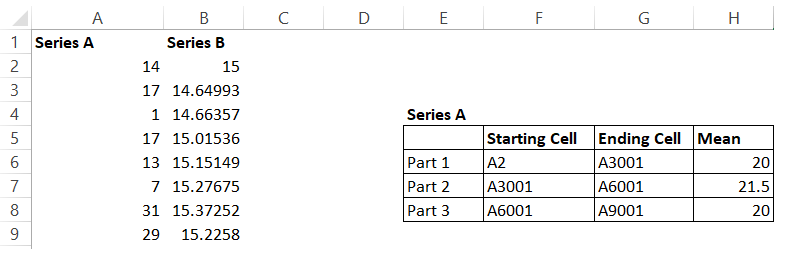Like I mentioned, I have 9000 data points in Series A and Series B. I have split Series A data points into 3 parts and as you can see, I’ve even highlighted the starting and ending cells for these parts.

The mean for all the three parts are similar, clearly satisfying the first condition.

I’ve done the same thing for Series B, here is how the mean looks –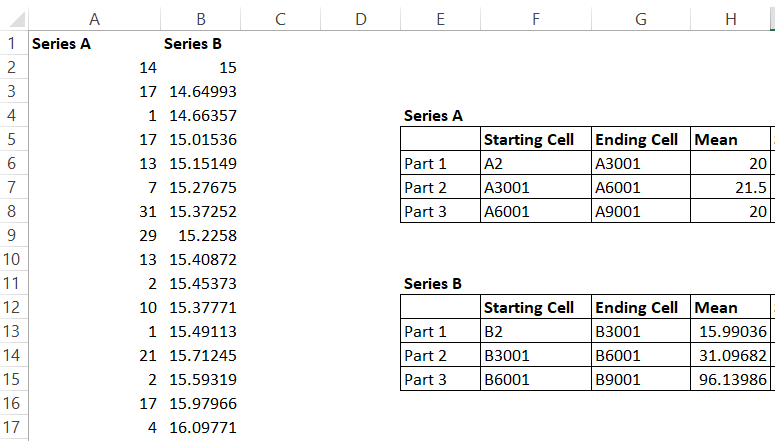Now as you can see, the mean for Series B swings quite wildly and thereby not satisfying the first condition for stationarity.

Condition 2 -The standard deviation should be within a range.

I’m following the same approach here – I will go ahead and calculate the standard deviation for all the three parts for both the series and observe the values.

Here is the result obtained for Series A –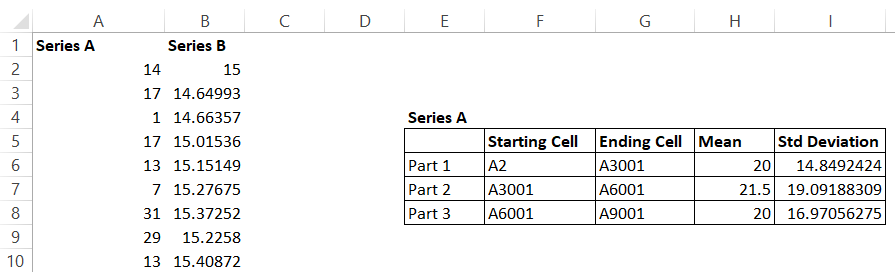The standard deviation oscillates between 14-19%, which is quite ‘tight’ and therefore qualifies the 2nd stationarity condition.

Here is how the standard deviation works out for Series B –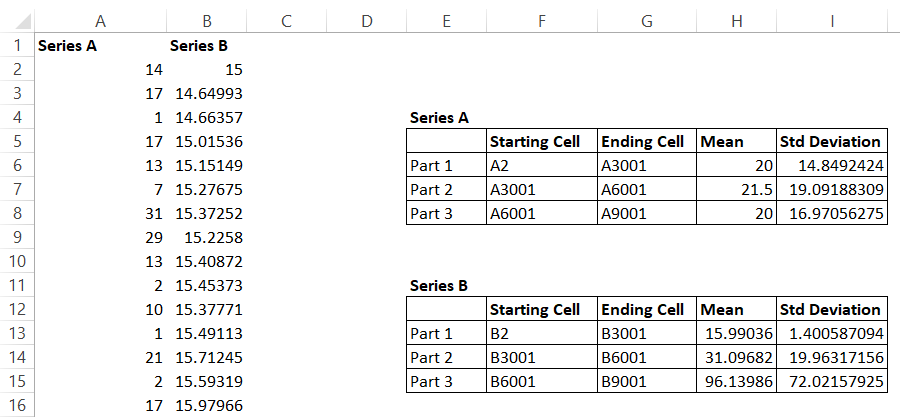Notice the difference? The range of standard deviation for Series B is quite random. Series B is clearly not a stationary series. However, Series A looks stationary at this point. However, we still need to evaluate the last condition i.e the autocorrelation bit, let us go ahead and do that.

Condition 3 – There should be no autocorrelation within the series

In layman words, autocorrelation is a phenomenon where any value in the time series is not really dependent on any other value before it.

For example, have a look at the snapshot below –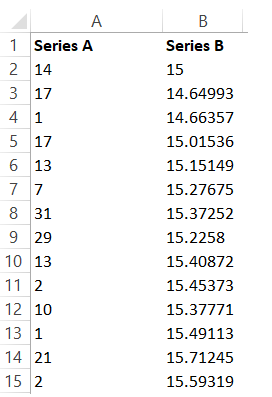The 9th value in Series A is 29, and if there is no autocorrelation in this series, the value 29 is not really dependent on any values before it i.e the values from cell 2 to cell 8.

But the question is how do we establish this?

Well, there is a technique for this.

Assume there are 10 data points, I take the data from Cell 1 to Cell 9, call this series X, now take the data from Cell 2 to Cell 10, call this Series Y. Now, calculate the correlation between Series X and Y. This is called 1-lag correlation. The correlation should be near to 0.

I can do this for 2 lag as well – i.e between Cell 1 to Cell 8, and then between Cell 3 to Cell 10, again, the correlation should be close to 0. If this is true, then it is safe to assume assumed that the series is not autocorrelated, and hence the 3rd condition for stationarity is proved.

I’ve calculated 2 lag correlation for Series A, and here is how it looks –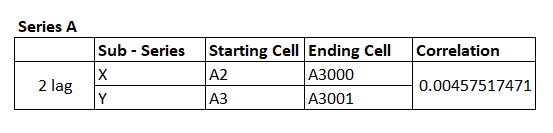Remember, I’m subdividing Series A into two parts and creating two subseries i.e series X and series Y. The correlation is calculated on these two subseries. Clearly, the correlation is close to zero and with this, we can safely conclude that Time Series A is stationary.

Let’s do this for Series B as well.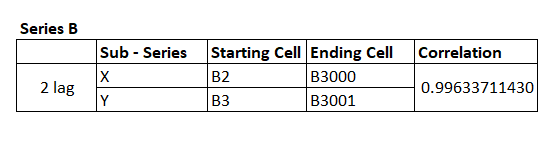I’ve taken a similar approach, and the correlation as you can see is quite close to 1.

So, as you can see all the conditions for stationarity is met for Series A – which means the time series is stationary. While Series B is not.

I know that I’ve taken a rather unconventional approach to explaining stationarity and co-integration. After all, no statistical explanation is complete without those scary looking formulas. But this is a deliberate approach and I thought this would be the best possible way to discuss these topics, as eventually, our goal is to learn how to pair trade efficiently and not really deep dive into statistics.

Anyway, you could be thinking if it is really required for you to do all of the above to figure out if the time series (residuals) are indeed stationary. Well, like I said before, this is not required.

We only need to look at the results of something called as the ‘The ADF Test’, to establish if the time series is stationary or not.

The augmented Dickey-Fuller or the ADF test is perhaps one of the best techniques to test for the stationarity of a time series. Remember, in our case, the time series in consideration is the residuals series.

Basically, the ADF test does everything that we discussed above, including a multiple lag process to check the autocorrelation within the series. Here is something you need to know – the output of the ADF test is not a definitive ‘Yes – this is a stationary series’ or ‘No – this is not a stationary series’. Rather, the output of the ADF test is a probability. It tells us the probability of the series, not being stationary.

For example, if the output of the ADF test a time series is 0.25, then this means the series has a 25% chance of not being stationary or in other words, there is a 75% chance of the series being stationary. This probability number is also called ‘The P value’.

To consider a time series stationary, the P value should be as low as 0.05 (5%) or lower. This essentially means the probability of the time series is stationary is as high as 95% (or higher).

Alright, so how do you run an ADF test?

Frankly, this is a highly complex process and unfortunately, I could not find a single source online which will help you run an ADF test for free. I do have an excel sheet (which has a paid plugin) to run an ADF test, but unfortunately, I cannot share it here. If I could, I would have.

If you are a programmer, I’ve been told that there are Python plugins easily available to run an ADF test, so you could try that.

But if you are a non-programmer like me, then you will be stuck at this stage. So here is what I will do, once in a weak or 15 days, I will try and upload a ‘Pair Data’ sheet, which will contain the following information of the best possible combination of pairs, this includes –

1. You will know which stock is X and which stock is Y
2. You will know the intercept and Beta of this combination
3. You will also know the p-value of the combination

The look back period for generating this is 200 trading days. I’ve restricted this just to banking stocks, but hopefully, I can include more sectors going forward. To help you understand this better, here is the snapshot of the latest Pair Datasheet for banking stocks –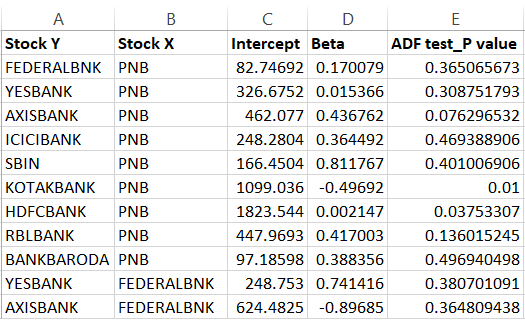The first line suggests that Federal Bank as Y and PNB as X is a viable pair. This also means, that the regression of Federal as Y and PNB as X and Federal as X and PNB as Y was conducted and the error ratio for both the combination was calculated, and it was found that Federal as Y and PNB as X had the least error ratio.

Once the order has been figured out (as in which one is Y and which one is X), the intercept and Beta for the combination has also been calculated. Finally, the ADF was conducted and the P value was calculated. If you see, the P value for Federal Bank as Y and PNB as X is 0.365.

In other words, this is not a combination you should be dealing with as the probability of the residuals being stationary is only 63.5%.

In fact, if you look at the snapshot above, you will find only 2 pairs which have the desired p-value i.e Kotak and PNB with a P value of 0.01 and HDFC and PNB with a P value of 0.037.

The p values don’t usually change overnight. Hence, for this reason, I check for p-value once in 15 or 20 days and try and update them here.

I think we have learned quite a bit in this chapter. A lot of information discussed here could be new for most of the readers. For this reason, I will summarize all the things you should know about Pair trading at this point –

1. The basic premise of pair trading
2. Basic overview of linear regression and how to perform one
3. In linear regression, we regress an independent variable, X against a dependent variable Y.
4. When we regress – some of the outputs that are of interest are the intercept, slope, residuals, standard error, and the standard error of the intercept
5. The decision to classify a stock as dependent and independent really depends on the error ratio.
6. We calculate the error ratio by interchanging both X and Y. The one which offers the lowest error ratio will define which stock is X and which on as Y
7. The residuals obtained from the regression should be stationary. If they are stationary, then we can conclude that the two stocks are co-integrated
8. If the stocks are cointegrated, then they move together
9. Stationarity of a series can be evaluated by running an ADF test.

If you are not clear on any of the points above, then I’d suggest you give this another shot and start reading from Chapter 7.

In the next chapter, we will try and take up an example of a pair trade and understand its dynamics.

You can download the Pair Data sheet, updated on 11th April 2018.

Lastly, this module (and this chapter, in particular) could not have been possible without the inputs from my good friend and an old partner, Prakash Lekkala. So I guess, we all need to thank him 🙂

### Key takeaways from this chapter –

1. If two stocks move together, then they are also cointegrated
2. You can pair trade on stocks which are cointegrated
3. If the residuals obtained from linear regression is stationary, then it implies the two stocks are co-integrated
4. A time series is considered stationary if the series has a constant mean, constant standard deviation, and no autocorrelation
5. The check for stationarity can be done by an ADF test
6. The p-value of the ADF test should be 0.05% or lower for the series to be considered stationary.

1.Deepu says:

Thanks Karthik.
Excellent writeup!!!
Is it possible to have the complete list of upcoming chapters to know where we are with regard this pair trade journey ?
Can we expect a few more chapters this month ? Sorry for being greedy…

Regards
Deepu

•Karthik Rangappa says:

I don’t plan for it in advance, but generally, go with the flow. To give you a rough idea, the next step would be to take up an example of a trade and try and put all the learning together. Hopefully, that will be exciting enough 🙂

•Deepu says:

Thanks Karthik.

Request you to share 5-6 examples so that it covers most of the leanings. Further what is the ADF plugin cost and where to buy it from ? Please share the details.

Regards
Deepak

•Akshay Hire says:

You can use R Studio package to run ADF test. There is a package called “urca” in r studio which enables this test.

•Karthik Rangappa says:

Thanks, Akshay. Yes, I’m aware R has a plugin, will have a look at URCA.

•Karthik Rangappa says:

The idea is to share a couple of live examples. Will share the other details as we progress.

•swapnil says:

Dear Karthik,

As you said I have stuck on ADF test. no friend with programming knowledge.
If I need to buy a paid plugin then how much it will cost?
Any other way to do the ADF test?

•Karthik Rangappa says:

Swapnil, unfortunately, I have not evaluated any paid versions. So cannot really comment. Thanks.

2.Anil Gowda says:

I’m glad to know new learning with your guidance. Seriously Its very educative and informative.
Thanks for Enlightenment us.

•Karthik Rangappa says:

Happy learning, Anil!

•Ar Arbit says:

I would say, it’s very addictive too along with being educative and informative.
Thanks a lot, Karthik.

•Karthik Rangappa says:

Glad to hear that, Arbit 🙂
Keep learning!

3.KM says:

Thanks prakash lekkala sir and karthik sir for your effort..

•Karthik Rangappa says:

Most welcome!

4.Muralidhar says:

Thank You Karthik sir,
Even though ADF test is not available , you have taught us how to calculate Stationarity using excel by dividing the data in to parts and calculate Mean,SD and 2 Lag correlation.But please mention how much variation in Mean,SD which would represent ‘p’value of 0.05 (rough estimate).

•Akshay Hire says:

You can run ADF test in R software, load package called “urca” in R. It’s really easy in R.

•swapnil says:

Dear Akshay,

•Sunil Balani says:

•ajay says:

deear sunil thanks a lot for the link please elaborate how to use it on excel? thanks in advance.

•Karthik Rangappa says:

For mean – I’d suggest a tight variation, not more than 3-5 points difference. For SD, technically you will have to look at the standard error of the standard deviation, but then, it may just get a little overboard. Stick to -5-10% at the most. This should result in a pvalue less than 0.05%.

5.Aditya says:

Can you please upload the PDF of all the chapters shared so far?
Thanks

•Karthik Rangappa says:

The modules will be completed to PDF once this is completed.